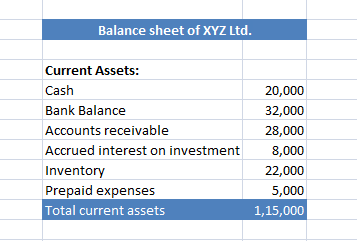# Liquid assets – Meaning, Formula & Example

Liquid assets mean those assets which can be converted into cash immediately without much loss.

The above definition seems to define current assets. So what’s the difference between these two?

One has to abstract the amount of inventories and prepaid expenses from the total amount of current assets to arrive at required figure.

### Formula for the calculation of Liquid Assets:

= Current assets – (Inventory + prepaid expenses)

Inventory and prepaid expenses are excluded from the scope of liquid assets because:

• Inventory can not be converted immediately into cash as and when required &
• Prepaid expenses cannot be converted into cash at all.

### Application of the formula:

Let’s suppose that XYZ Ltd has following figures on its balance sheet under the head ‘Current assets’Using the formula and figures, we can calculate the total current assets as follows:

 Total current assets 1,15,000 Total amount of ‘Inventory’ and ‘Prepaid expenses’ 22,000 + 5,000 = 27,000

Liquid assets = 1,15,000 – 27,000 = 88,000.

Posted on Categories Dictionary

## One thought on “Liquid assets – Meaning, Formula & Example”

1.Delores says: